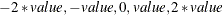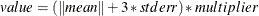The ENTROPY Procedure (Experimental)

PRIORS Statement

• PRIORS variable <support points < (priors) >> variable <support points < (priors) >> …;

The PRIORS statement specifies the support points and prior weights for the coefficients on the variables.

Support points for coefficients default to five points, determined as follows:where value is computed aswhere the mean and the stderr are obtained from OLS and the multiplier depends on the MULTIPLIER= option. The MULTIPLIER= option defaults to 2 for unrestricted models and to 4 for restricted models. The prior probabilities for each support point default to the uniform distribution.

The number of support points must be at least two. If priors are specified, they must be positive and there must be the same number of priors as there are support points. Priors and support points can also be specified through the PDATA= data set.